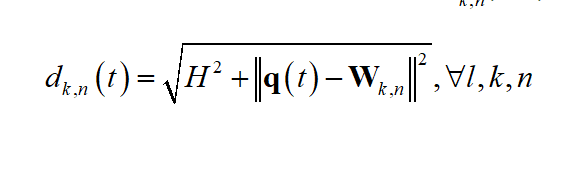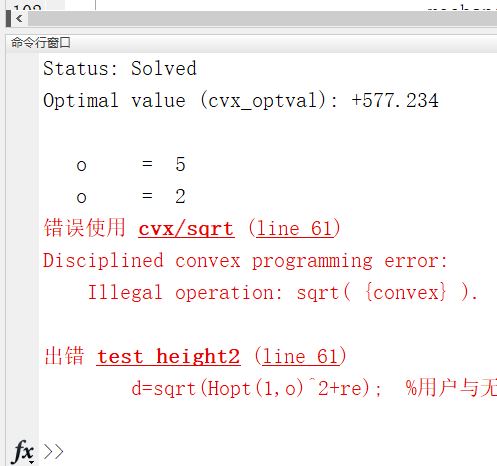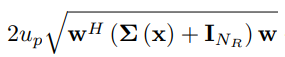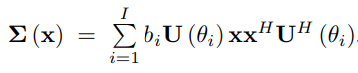# Why the sqrt({convex}) is wrong?

I don’t know why it is wrong when I use sqrt({convex}).

There are my codes:

``````Hmax=500;
H=zeros(1,Omax);
H(:,o)=(0.2*Hmax);
tempRate=zeros(48,1);
while 1
cvx_begin
variable Hopt(1,Omax)
expression tempRate(48,1)
expression f
for i=1:length(q)
a=q(i,:);
for j=1:U
re2(j+(i-1)*U)=sum((a-w(j)).^2 ,2);
end
end
re=re2';
**d=sqrt(Hopt(1,o)^2+re);**
``````

This is my equation:Use `norm` because `sqrt(convex)` is not allowed in CVX.

`d=norm([Hopt(1,o),sqrt(re)]);`

You might be able to do what you want with `norm(suitable vector)` without using all those for loops, but I’m not clear on the dimensions or everything, so I’ll leave those details to you.

help cvx/sqrt
Discipined convex programming information for sqrt:
sqrt(X) is log-concave and nondecreasing in X. Therefore, when used
in DCPs, X must be concave (or affine).

``````Disciplined geometric programming information for sqrt:
sqrt(X) is log-log-affine and nondecreasing in X. Therefore, when
used in DGPs, X may be log-affine, log-convex, or log-concave.``````

Thank you very much for your help! I am trying it.

I try to use this method to solve the following problems：but the following errors：

what can I do to solve it?

I’m not sure this is right. So I leave you to check this and fix it up.
`sqrt(w'*b*(U*x*x'*U'+eye(N))*w = (sqrt(b)*x'*U'*w)'*(sqrt(b)*x'*U'*w) + w'*w) = norm([sqrt(b)*x'*U'*w;w])`

it it can be done, I believe you will need to do this or sometthing similar, using norm.

OK，that is solved.Thanks! Mark